# JP4112849B2 - Semiconductor memory device - Google Patents

## Info

Publication number
JP4112849B2
JP4112849B2 JP2001356571A JP2001356571A JP4112849B2 JP 4112849 B2 JP4112849 B2 JP 4112849B2 JP 2001356571 A JP2001356571 A JP 2001356571A JP 2001356571 A JP2001356571 A JP 2001356571A JP 4112849 B2 JP4112849 B2 JP 4112849B2
Authority
JP
Japan
Prior art keywords
circuit
data
bit
bits
error
Prior art date
Legal status (The legal status is an assumption and is not a legal conclusion. Google has not performed a legal analysis and makes no representation as to the accuracy of the status listed.)
Active
Application number
JP2001356571A
Other languages
Japanese (ja)
Other versions
JP2003157697A (en
Inventor

Original Assignee

Priority date (The priority date is an assumption and is not a legal conclusion. Google has not performed a legal analysis and makes no representation as to the accuracy of the date listed.)
Filing date
Publication date
Application filed by 株式会社東芝 filed Critical 株式会社東芝
Priority to JP2001356571A priority Critical patent/JP4112849B2/en
Publication of JP2003157697A publication Critical patent/JP2003157697A/en
Application granted granted Critical
Publication of JP4112849B2 publication Critical patent/JP4112849B2/en
Application status is Active legal-status Critical
Anticipated expiration legal-status Critical

## Images

•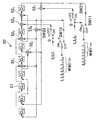•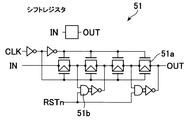•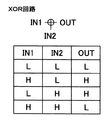•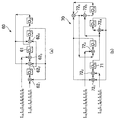•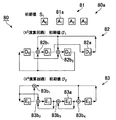•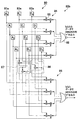••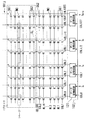••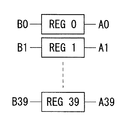•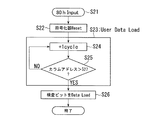•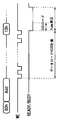•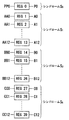•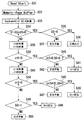•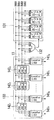•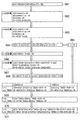•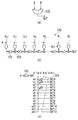•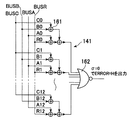•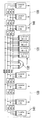•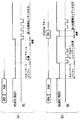•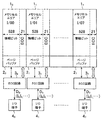•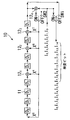••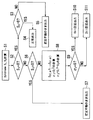•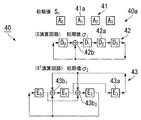•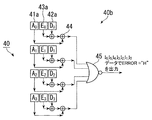## Classifications

• HELECTRICITY
• H03BASIC ELECTRONIC CIRCUITRY
• H03MCODING; DECODING; CODE CONVERSION IN GENERAL
• H03M13/00Coding, decoding or code conversion, for error detection or error correction; Coding theory basic assumptions; Coding bounds; Error probability evaluation methods; Channel models; Simulation or testing of codes
• H03M13/65Purpose and implementation aspects
• H03M13/6566Implementations concerning memory access contentions
• GPHYSICS
• G06COMPUTING; CALCULATING; COUNTING
• G06FELECTRIC DIGITAL DATA PROCESSING
• G06F11/00Error detection; Error correction; Monitoring
• G06F11/07Responding to the occurrence of a fault, e.g. fault tolerance
• G06F11/08Error detection or correction by redundancy in data representation, e.g. by using checking codes
• G06F11/10Adding special bits or symbols to the coded information, e.g. parity check, casting out 9's or 11's
• G06F11/1008Adding special bits or symbols to the coded information, e.g. parity check, casting out 9's or 11's in individual solid state devices
• G06F11/1068Adding special bits or symbols to the coded information, e.g. parity check, casting out 9's or 11's in individual solid state devices in sector programmable memories, e.g. flash disk
• HELECTRICITY
• H03BASIC ELECTRONIC CIRCUITRY
• H03MCODING; DECODING; CODE CONVERSION IN GENERAL
• H03M13/00Coding, decoding or code conversion, for error detection or error correction; Coding theory basic assumptions; Coding bounds; Error probability evaluation methods; Channel models; Simulation or testing of codes
• H03M13/03Error detection or forward error correction by redundancy in data representation, i.e. code words containing more digits than the source words
• H03M13/05Error detection or forward error correction by redundancy in data representation, i.e. code words containing more digits than the source words using block codes, i.e. a predetermined number of check bits joined to a predetermined number of information bits
• H03M13/13Linear codes
• H03M13/15Cyclic codes, i.e. cyclic shifts of codewords produce other codewords, e.g. codes defined by a generator polynomial, Bose-Chaudhuri-Hocquenghem [BCH] codes
• H03M13/151Cyclic codes, i.e. cyclic shifts of codewords produce other codewords, e.g. codes defined by a generator polynomial, Bose-Chaudhuri-Hocquenghem [BCH] codes using error location or error correction polynomials
• H03M13/152Bose-Chaudhuri-Hocquenghem [BCH] codes
• HELECTRICITY
• H03BASIC ELECTRONIC CIRCUITRY
• H03MCODING; DECODING; CODE CONVERSION IN GENERAL
• H03M13/00Coding, decoding or code conversion, for error detection or error correction; Coding theory basic assumptions; Coding bounds; Error probability evaluation methods; Channel models; Simulation or testing of codes
• H03M13/03Error detection or forward error correction by redundancy in data representation, i.e. code words containing more digits than the source words
• H03M13/05Error detection or forward error correction by redundancy in data representation, i.e. code words containing more digits than the source words using block codes, i.e. a predetermined number of check bits joined to a predetermined number of information bits
• H03M13/13Linear codes
• H03M13/15Cyclic codes, i.e. cyclic shifts of codewords produce other codewords, e.g. codes defined by a generator polynomial, Bose-Chaudhuri-Hocquenghem [BCH] codes
• H03M13/151Cyclic codes, i.e. cyclic shifts of codewords produce other codewords, e.g. codes defined by a generator polynomial, Bose-Chaudhuri-Hocquenghem [BCH] codes using error location or error correction polynomials
• H03M13/158Finite field arithmetic processing

## Description


BACKGROUND OF THE INVENTION
The present invention relates to a semiconductor memory device such as a NAND flash memory, and more particularly to a semiconductor memory device having an error correction function in a chip.

[Prior art]
It is known that NAND-type flash memory has cell characteristics that deteriorate when rewriting is repeated and that data becomes garbled when left for a long time. Therefore, in order to increase the reliability of this type of NAND flash memory, a semiconductor memory in which an ECC (Error Correcting Code) circuit for error detection and correction is mounted in the chip has been proposed (for example, specially).Open 2000-348497, specialOpen 2001-14888 etc.).

FIG. 21 is a block diagram schematically showing a configuration of a conventional NAND flash memory equipped with an ECC circuit.
This memory has 8 memory cell areas 10, 11, ..., 17It is composed of Each memory cell area 10~ 17Is composed of a plurality of memory cells (not shown) arranged in a matrix, and 528 bits of data (= 1 page) through 528 bit lines for 528 memory cells connected to a common word line. Can be collectively written and read. Each memory cell area 10~ 17Includes a page buffer 2 that holds 528 bits of write data and read data.0~ 27Is connected. Page buffer 20~ 27And each memory cell area 10~ 17I / O terminal 4 provided for each0~ 47Between each memory cell area 10~ 17ECC circuit 3 every time0~ 37Are provided.

Each ECC circuit 30~ 37Indicates each memory cell area 10~ 17An encoding function for adding a predetermined number of check bits (ECC) to one page (528 bits) of information bits stored in the memory, and detecting a predetermined number of bits from the information bits to which the check bits are added, and For example, a BCH (Bose-Chaudhuri-Hocquenghem) code capable of correcting a plurality of bits with a relatively small circuit scale is used as an error correction code. Between the outside and the memory, for example, data is read / written in units of 8 bits corresponding to the number of memory cells. Therefore, each ECC circuit 30~ 37The data is input bit by bit, and the internal cyclic shift register is cycled bit by bit and output bit by bit, whereby the encoding process and the decoding process are executed.

Next, a conventional ECC circuit 3 using a BCH code0~ 37The encoding and decoding operations will be described.
Note that the number of check bits of a BCH code that performs 528 bits of information and performs 2-bit error correction and 3-bit error detection is 21 bits, but here, for simplicity of explanation, the number of information bits k = 7, the code length An error detection / correction method using a BCH code capable of 2-bit error correction and 3-bit error detection with n = 15 and the number of correction bits t = 2 will be described as an example.
It is generally known that a generator polynomial necessary for encoding and decoding in this case is as follows.

[Expression 1]

(1) Encoding circuit
FIG. 22 is a block diagram showing an encoding circuit 10 functionally configured inside a conventional ECC circuit 3i (i = 0, 1,..., Or 7). The encoding circuit 10 includes a register D7, D6, DFive, DFour, DThree, D2, D1, D0Shift register 11 and XOR circuit 12 for modulo 2 operation1, 122, 12Three, 12FourAnd changeover switches SW1 and SW2.

The operation of moving the shift register 11 once corresponds to multiplying the value of the shift register 11 by X times. Now, the value of the data stored in the shift register 11 is expressed as follows.

[Expression 2]
a0X0+ A1X1+ A2X2+ AThreeXThree+ AFourXFour+ AFiveXFive+ A6X6+ A7X7
[However, aiIs register DiThe value stored ini= 0 or 1 (i = 0 to 7)]

By shifting this once,

[Equation 3]
a0X1+ A1X2+ A2XThree+ AThreeXFour+ AFourXFive+ AFiveX6+ A6X7+ A7X8

It becomes. From the generator polynomial G (x) in Equation 1, X8= X7+ X6+ XFourSince there is a +1 relationship, Equation 3 is

[Expression 4]
a7X0+ A0X1+ A1X2+ A2XThree+ (AThree+ A7) XFour+ AFourXFive+ (AFive+ A7) X6+ (A6+ A7) X7

It can be expressed as This is because register D is shifted when each bit is shifted.7Value a7Register D0And register DThree, D7Value aThree, A7XOR circuit 121Add register DFourAnd register DFive, D7Value aFive+ A7XOR circuit 122Add register D6And register D6, D7Value a6+ A7XOR circuit 12ThreeAdd register D7It is equivalent to storing in.

At the time of encoding, first, the switches SW1 and SW2 are connected to the ON side, and input data (information bits) I from the outside via the I / O terminal 4i.0, I1, I2, IThree, IFour, IFive, I6(I0~ I6= 0 or 1) is input bit by bit. Input data I0~ I6Each time 1 bit is input, the shift register 11 operates once. Data I0~ I6Since the switch SW1 is ON while is input, these data are output to the page buffer 2i bit by bit as they are. At the same time, data I0~ I6XOR circuit 121In register D7Value a7And are sequentially stored in the shift register 11. Data I0~ I6When the input to the page buffer 2i ends, the register D of the shift register 117, D6, DFive, DFour, DThree, D2, D1, D0Inside is a check bit I7, I8, I9, ITen, I11, I12, I13, I14Are stored. Thereafter, the switches SW1 and SW2 are both connected to the OFF side, and each time the shift register 11 operates, the check bit I is passed through the switch SW1.7~ I14Are serially output to the page buffer 2i. Then, the information bits and check bits stored in the page buffer 2i are stored in the memory cell area 1i. At the same time, the value of the shift register 11 is reset.

(2) Decoding circuit
Next, the decoding circuit will be described. The decoding circuit includes a syndrome calculation circuit and an error position detection circuit. In the case of 2-bit error correction, two syndromes S are used for decoding.1, SThreeAre required and these are the minimum polynomial M1(X) = XFourIt is known to be obtained by + X + 1. FIG. 23A shows a conventional S.1A diagram specifically showing the syndrome calculation circuit 20 and FIG.Three2 is a diagram illustrating a syndrome calculation circuit 30. FIG.

S in FIG.1The syndrome calculation circuit 20 calculates the minimum polynomial M1Based on (x), register DThree, D2, D1, D0Shift register 21 and XOR circuit 221, 222It is comprised by. Here, the operation of moving the shift register 21 once corresponds to multiplying the value of the shift register 21 by X times. Now, the value stored in the shift register 21 is expressed as follows.

[Equation 5]
a0X0+ A1X1+ A2X2+ AThreeXThree
[However, aiIs register DiThe value stored ini= 0 or 1 (i = 0 to 3)]

By shifting this once

[Formula 6]
a0X1+ A1X2+ A2XThree+ AThreeXFour

The minimum polynomial M of α1X from (x)Four= X + 1 because there is a relationship

[Expression 7]
aThreeX0+ (A0+ AThree) X1+ A1X2+ A2XThree

It becomes. This is because register D is shifted when each bit is shifted.ThreeValue aThreeRegister D0And register D0, DThreeValue a0, AThreeXOR circuit 122Add register D1It is equivalent to storing in. Information bit I stored in the memory cell0~ I6And inspection bit I7~ I14Means S in this order1One bit at a time is input to the syndrome calculation circuit 20, and each time one bit is input, the shift register 21 operates once. All bits I0~ I14Is input, the shift register 21 (D0~ DThree) Syndrome S1Is generated.

S in FIG.ThreeThe syndrome calculation circuit 30 is also S1D as in the syndrome calculation circuit 20Three, D2, D1, D0Shift register 31 and XOR circuit 32 comprising1, 322, 32Three, 32FourAnd the minimum polynomial M1X of (x)ThreeIt is constituted by a circuit. SThreeIn the syndrome calculation circuit 30, the operation of moving the shift register 31 once converts the value of the shift register 31 to XThreeIt is equivalent to doubling. Now, the value stored in the shift register 31 is expressed as in Expression 5, and this is expressed as XThreeThen

[Equation 8]
a0XThree+ A1XFour+ A2XFive+ AThreeX6

The minimum polynomial M of α1X from (x)Four= X + 1 because there is a relationship

[Equation 9]
a1X0+ (A1+ A2) X1+ (A2+ AThree) X2+ (A0+ AThree) XThree

It becomes. This is because register D is shifted when each bit is shifted.1Value a1Register D0And register D1, D2Value a1, A2XOR circuit 322Add register D1And register D2, DThreeValue a2, AThreeXOR circuit 32ThreeAdd register D2And register D0, DThreeValue a0, AThreeXOR circuit 32FourAdd register DThreeIt is equivalent to storing in. This SThreesyndromeCalculationThe circuit 30 also has an information bit I stored in the memory cell.0~ I6And inspection bit I7~ I14Are input one bit at a time in this order, and the shift register 31 operates once each time one bit is input. All bits I0~ I14Is input, the shift register 31 (D0~ DThree) Syndrome SThreeIs generated.

FIG. 24 is a flowchart showing an algorithm of the decoding process. First, S1, S2In the syndrome calculation circuits 20 and 30, the syndrome S is calculated from the information bit and the check bit read from the memory cell area 1i.1, SThreeIs calculated (step S1). Syndrome S1, SThreeBut S1= SThreeWhen = 0, the information bits read out as having no error are output as they are (steps S2, S3, S4). Syndrome S1, SThreeWhen only one of them is 0, correction is impossible and the data is output as it is (steps S2, S3, S5, S6, S7). S1≠ 0, SThreeIf ≠ 0, calculate and σ1= S1 2, Σ2= S1 Three+ SThree(Steps S2, S6, S8). Where σ2If = 0 (step S9), it is found that there is a 1-bit error, so 1-bit correction is performed and output (step S10). σ2If ≠ 0 (step S9), it can be seen that there is a 2-bit error, so 2-bit correction is performed and output (step S11).

The position of the error bit is expressed by Z = α in the error position polynomial σ (Z) shown in Equation 10.IIt is generally known that it is obtained by sequentially substituting (I = 0, 1, 2, 3, 4, 5, 6). σ (αI) = 0, i is the error position.

[Expression 10]
σ (Z) = S1+ Σ1× Z + σ2× Z2

The configuration of an error position detection circuit configured based on such points is shown in FIGS. FIG.1, Σ1And σ2FIG. 26 shows a first calculation unit 40a that calculates and stores the first calculation unit 40a, and FIG. 2 shows a calculation unit 40b. As shown in FIG. 25, the first arithmetic unit 40a includes a shift register 41, an X arithmetic circuit 42, and an X arithmetic circuit 42.2An arithmetic circuit 43 is used. The shift register 41a has a syndrome S.1Is stored in the shift registers 42a and 43a.1= S1 2, Σ2= S1 Three+ SThreeIs stored. Here, the value of the shift register 42a is set to

[Expression 11]
a0X0+ A1X1+ A2X2+ AThreeXThree
[However, aiIs register DiThe value stored ini= 0 or 1 (i = 0 to 3)]

Then, since the X arithmetic circuit 42 multiplies this by X, the value of the shift register 42a is

[Expression 12]
a0X1+ A1X2+ A2XThree+ AThreeXFour

The minimum polynomial M of α1X from (x)Four= X + 1 because there is a relationship,

[Formula 13]
aThreeX0+ (A0+ AThree) X1+ A1X2+ A2XThree

It becomes. This is because register D is shifted when each bit is shifted.ThreeValue aThreeRegister D0And register D0, DThreeValue a0, AThreeIs added by the XOR circuit 42b to register D1It is equivalent to storing in.

X2The arithmetic circuit 43 converts the value of the shift register 43a to X2Therefore, if the value shown in Equation 11 is stored in the value of the shift register 43a, this is expressed as X2By multiplying, the value of the shift register 43a becomes

[Expression 14]
a0X2+ A1XThree+ A2XFour+ AThreeXFive

The minimum polynomial M of α1X from (x)Four= X + 1 because there is a relationship,

[Expression 15]
a2X0+ (A2+ AThree) X1+ (A0+ AThree) X2+ A1XThree

It becomes. This is because register E is shifted when each bit is shifted.2Value a2Register E0And register E1Value a1Register EThreeAnd register E2, EThreeValue a2, AThreeXOR circuit 43b1Register E1And register E0, EThreeValue a0, AThreeXOR circuit 43b2Register E2It is equivalent to storing in.

1-bit data I0~ I6When the shift register 41a, 42a, 43a is operated once at the time of1Is multiplied by Z and X2In the arithmetic circuit 43, σ2Is the term Z2Doubled. In the NAND flash memory, the shift registers 41a, 42a, and 43a are moved in synchronization with a toggle signal when the information bit stored in the memory cell is output to the outside of the chip. As a result, in the second calculation unit 40b, the output of the result calculated by the XOR circuit 44 and the NOR gate 45 becomes 1 when the error position is reached. Detection and correction are performed.

[Problems to be solved by the invention]
As described above, in the conventional ECC circuit using the BCH code, one shift and calculation for a 1-bit input is a basic operation. In the NAND flash memory, data is input from the outside in units of 8 IO or 16 IO for one address, so that error correction is performed for each IO or 8 or 16 times during this one input. Must be calculated. However, performing 8 or 16 calculations during one input means that only this part is operated at high speed, and a special process is required, so that it cannot be performed in reality.

Therefore, conventionally, an ECC circuit 3i is provided for each memory cell area 1i (each I / O) to perform error correction in units of each memory cell area 1i. In this case, in the NAND flash memory, data is read or programmed in units of one page (528 bytes), so if 2-bit error correction 3-bit error detection is performed for each I / O, the number of information bits is 528 bits and the number of inspection bits are 21 bits, and a total of 21 × 8 = 168 bits of extra inspection bits are required for the entire chip. This has been an impediment to increasing the integration density of the chip.

The present invention has been made in view of such problems, and an object of the present invention is to provide a semiconductor memory device that can reduce the number of inspection bits with respect to the number of information bits and improve the integration degree of the chip.

[Means for Solving the Problems]
The present invention provides a plurality of memory cell areas in which a plurality of memory cells are arranged in a matrix, and write data to the memory cell area and read data from the memory cell area provided in a data input / output section of each memory cell area. A plurality of input / output terminals for inputting data for writing to each memory cell area from the outside and outputting data read from each memory cell area to the outside, An encoder that is provided between a plurality of input / output terminals and the plurality of buffers, and generates and adds an error correction check bit to data to be written to the plurality of memory cell areas, and the generated check An error is provided with a decoder that performs error correction processing on data read from the plurality of memory cell areas using bits. In the semiconductor memory device including the correction circuit, when the number of bits of data (however, a check bit is not included) serving as a unit of writing and reading with respect to one memory cell area is M, M × N bits (N is an integer of 2 or more) is used as an information bit length, one check bit consisting of a plurality of bits is assigned, and at least one of encoding processing and decoding processing is executed by processing N-bit data in parallel. The encoder includes a shift register that generates, as check bits, a cyclic code derived from a generator polynomial G (X) defined by an information bit length k = M × N, a code length n, and a correction bit length t. Comprising an arithmetic circuit, the shift register inputs A-bit data (A is an integer of 2 or more) having different orders in parallel, and performs one shift operation. In each of the data XAThe check bit is generated internally by M × N / A shift operations.The plurality of buffers load the data in units of A bits in synchronization with the shift operation of the shift register, and load the generated check bits when the M × N / A shift operations are completed, Writing the loaded data and check bits into the plurality of memory cell areasIt is characterized by that.

According to the present invention, information bits are conventionally generated for each M bits, which is a unit of access to each memory cell area. However, since N bits can be processed in parallel, M × N bits Thus, one check bit can be assigned to the total number of check bits, and the number of check bits for the total information bits can be reduced. As a result, the integration degree of the chip can be improved while mounting the error correction circuit.
As an example, in a t-bit correction (t + 1) bit error detection BCH code,

[Expression 16]
Code length n = 2m-1
Number of information bits k ≦ 2m−1−m × t
Number of inspection bits mt + 1 ≦ n−k
Number of correction bits t

Therefore, if the number of m is increased, the code length and the number of information bits increase exponentially, but the number of check bits does not increase so much. For this reason, the number of information bits is 2mThe ratio of the number of check bits to the number of information bits becomes smaller as it is larger as long as it does not exceed −1−m × t. Therefore, it is advantageous to provide check bits for as many information bits as possible.
For example, a NAND flash memory reads or programs in one page (528 bytes), but when 2-bit error correction and 3-bit error detection are performed for each IO (each memory cell area), the number of information bits is k = Since 528 bits and the number of check bits are 21 bits, a total of 21 × 8 = 168 extra check bits are required.
However, when 2-bit error correction and 3-bit error detection are performed in one unit for one page, when the number of information bits is 4224 bits, the number of check bits may be 27 bits. Even for 3-bit error correction and 4-bit error detection, when the number of information bits is 4224 bits, the number of check bits is 40 bits.

In one embodiment of the present invention, N memory cell areas are provided. The encoder is composed of, for example, a shift register and an arithmetic circuit that generate a cyclic code derived from a generator polynomial G (X) defined by an information bit length k = M × N, a code length n, and a correction bit length t as check bits. , The shift register inputs N-bit data having different orders in order, and inputs each data in one shift operation.NThe check bit is generated internally by M times of shift operations.

The decoder also includes, for example, a syndrome calculation circuit that calculates a syndrome by inputting the information bits and check bits, a first calculation unit that calculates a term of an error locator polynomial from the calculated syndrome, and a calculation An error position detection circuit comprising a second calculation unit for detecting an error position by calculating an error position polynomial from an error polynomial term, and the detection for the data read from the memory cell area via a buffer. A data inversion circuit that performs data inversion processing at the error position, and the syndrome calculation circuit has a minimum polynomial M of an α operator defined by an information bit length k = M × N, a code length n, and a correction bit length t. X) based on a shift register that generates a cyclic code as a syndrome and an arithmetic circuit, and the shift register has N bits with different orders. Input data in parallel, and each data is X in one shift operation.KN(K is an integer) is multiplied and all the information bits and check bits are input to generate a syndrome inside.

The error position detection circuit includes a cyclic code based on a minimum polynomial M (X) of an α operator defined by an information bit length k = M × N, a code length n, and a correction bit length t. Is generated as a term of an error position polynomial, and the shift register performs a shift operation in synchronization with the data output from the memory area, and outputs each data to XKThe error correction position is sequentially detected by multiplying (K is an integer).

The encoder, the syndrome calculation circuit, and the first arithmetic unit may be configured by switching between a register and an arithmetic circuit constituting the arithmetic logic circuit. The decoder may further include a Galois arithmetic circuit used when calculating a syndrome or error locator polynomial term. In addition, the second arithmetic unit, for example, inserts N pieces of locators provided for each of the N-bit data and the adjacent locators into X data.LX to doubleLAnd an arithmetic circuit (L is an integer).

DETAILED DESCRIPTION OF THE INVENTION
Hereinafter, embodiments of the present invention will be described with reference to the drawings.
(1) First embodiment
First, in order to understand the present invention, an example of 2-bit error correction with the number of information bits k = 7, the code length n = 15, and the number of correction bits t = 2 will be described as the first embodiment.

(1-1) Coding circuit
In the conventional encoding circuit 10 shown in FIG.0 EnteredInput data I0XOR circuit 12FourX of the encoding circuit7Is added to the term, and then multiplied by X. Since the value of each register 11 of the encoding circuit 10 is 0 in the initial state, if this value is (0)

[Expression 17]
(0 + I0X7) X

It becomes. Next, input data I1Is input to the encoding circuit 10, the input data I1X of the encoding circuit 107Since it is added to the term of this, and is multiplied by X after this,

[Expression 18]
((0 + I0X7) X + I1X7) X

Next, input data I2Is input to the encoding circuit 10, the input data I2X of the encoding circuit 107Since it is added to the term of this, and is multiplied by X after this,

[Equation 19]
(((0 + I0X7) X + I1X7) X + I2X7) X

It becomes.
Similarly, input data I6Are input to the encoding circuit 10 until

[Expression 20]
(((((((0 + I0X7) X + I1X7) X + I2X7) X + IThree  X7) X + IFourX7) X + IFiveX7) X + I6X7) X

It becomes. If this equation is transformed

[Expression 21]
((((0 + I0X7+ I1X6) X2+ I2X7+ IThreeX6) X2+ IFourX7+ IFiveX6) X2+ I6X7) X

This is the input data I0, I1Are X of the encoding circuit 10 respectively.7, X6After that, add X2Then the input data I2, IThreeAre X of the encoding circuit 10 respectively.7, X6After that, add X2Then the input data IFour, IFiveAre X of the encoding circuit 10 respectively.7, X6After that, add X2It means being doubled. In other words, after 2-bit input, data is transferred to X by one operation of the shift register 11.2Doubled. However, the last I6Is a 1-bit input as usual and is multiplied by X.

Here, it is assumed that the value of the shift register 11 is the value represented by Equation 2, and this is X2When doubled

[Expression 22]
a0X2+ A1XThree+ A2XFour+ AThreeXFive+ AFourX6+ AFiveX7+ A6X8+ A7X9

From the generator polynomial G (x) of Equation 1, X8= X7+ X6+ XFourSince there is a +1 relationship,

[Expression 23]
(A6+ A7) X0+ A7X1+ A0X2+ A1XThree+ (A2+ A6+ A7) XFour+ (AThree+ A7) XFive+ (AFour+ A6+ A7) X6+ (AFive+ A6) X7

It becomes.

FIG. 1 is a block diagram showing a configuration of an encoding circuit 50 according to this embodiment in which Equation 23 is specifically configured by a circuit.
The encoding circuit 50 includes a register D7, D6, DFive, DFour, DThree, D2, D1, D0Shift register 51 and XOR circuit 521, 522, 52Three, 52Four, 52Five, 526, 527And four switches SW11, SW12, SW21, SW22 for switching input data and output data. As shown in FIG. 2, the shift register 51 is a four-stage transfer in which the data content is reset by a reset signal RSTn and 1-bit data is transferred from the input terminal IN to the output terminal OUT in synchronization with the clock signal CLK. It consists of a gate 51a and a necessary gate circuit 51b. As shown in FIG. 3, the XOR circuit 52 performs a modulo 2 operation on the data input to the input terminals IN1 and IN2, and outputs the result from the output terminal OUT.

The encoding circuit 50 performs the register D in one shift operation based on Equation 23.6, D7Value a6, A7XOR gate 526Add register D0And register D7Value a7Register D1And register D0Value a0Register D2And register D1Value a1Register DThreeAnd register D2, D6, D7Value a2, A6, A7XOR circuit 521, 526Add register DFourAnd register DThree, D7Value aThree, A7XOR circuit 522Add register DFiveAnd register DFour, D6, D7Value aFour, A6, A7XOR circuit 52Three, 526Add register D6And register DFive, D6Value aFive, A6XOR circuit 52FiveAdd register D7To store.

Input data (information bit) I input from the outside because it is written to the memory0, I1, I2, IThree, IFour, IFive, I6Is the input data I0, I2, IFourAnd input data I1, IThree, IFiveInput data I0, I2, IFourIs input to the ON terminal of the switches SW11 and SW21, and the input data I1, IThree, IFiveIs input to the ON-side terminals of the switches SW12 and SW22. In other words, the input data is 2 bits at a time (I0, I1), (I2, IThree), (IFour, IFive) In parallel, and the shift register 51 operates once after the input. However, since every other shift register 51 is connected, the data is transferred to X by one shift operation.2Doubled. Data (I0, I1), (I2, IThree), (IFour, IFive) Is input, the switches SW11, SW21, SW12, and SW22 are all ON, and these data are output in parallel by 2 bits as they are. At the same time, data I0, I2, IFourXOR circuit 527In register D7Value a7And sequentially stored in the shift register 51, the data I1, IThree, IFiveXOR circuit 52FourIn register D7Value a7And are sequentially stored in the shift register 51. However, the last I of the input data6Is a 1-bit input, so that it is switched to the same connection state as the conventional encoding circuit 10 shown in FIG. Such switching is performed because k = 7 is selected as the number of information bits. Data I0, I1, I2, IThree, IFour, IFive, I6Is finished, register D of shift register 517, D6, DFive, DFour, DThree, D2, D1, D0Inside is a check bit I7, I8, I9, ITen, I11, I12, I13, I14Are stored. Thereafter, the switches SW11, SW21, SW12, and SW22 are all connected to the OFF side, and each time the shift register 51 is operated, the check bit I7, I9, I11, I13Is output, and the check bit I is output to the output of SW12.8, ITen, I12, I14Is output. At the same time, the value of the shift register 51 is reset. As a result, it is possible to generate check bits by parallel processing of 2-bit input.

(1-2) Decoding circuit
▲ 1 ▼ S1Syndrome calculation circuit
Conventional S in FIG.1In the syndrome calculation circuit 20, first, S1After the value in the syndrome calculation circuit 20 is multiplied by X, the XOR circuit 221X0Input data I0Is added. Initially S1Since the value of the shift register 21 of the syndrome calculation circuit 20 is 0, if this value is (0),

[Expression 24]
0xX + I0

It becomes. Then S1After the value in the syndrome calculation circuit 20 is multiplied by X, X0Input data I1Will be added,

[Expression 25]
(0xX + I0) X + I1

It becomes. Followed by S1After the value in the syndrome calculation circuit 20 is multiplied by X, X0Input data I2Will be added,

[Equation 26]
((0 × X + I0) X + I1) X + I2

It becomes. In this way, the input data I14Until S1When input to the syndrome calculation circuit 20,

[Expression 27]
((((((((((((0 × X + I0) X + I1) X + I2) X + IThree) X + IFour) X + IFive) X + I6) X + I7) X + I8) X + I9) X + ITen) X + I11) X + I12) X + I13) X + I14

It becomes. If this equation is transformed,

[Expression 28]
(((((((0 × X2+ I0X + I1) X2+ I2X + IThree) X2+ IFourX + IFive) X2+ I6X + I7) X2+ I8X + I9) X2+ ITenX + I11) X2+ I12X + I13) X + I14

And this is S1The value in the syndrome calculation circuit 20 is X2After doubling, the input data I0, Is X1Term, input data I1Is X0Is added to each term, and then S1The value in the syndrome calculation circuit 20 is X2After doubling, the input data I2, Is X1Term, input data IThreeIs X0Is added to each term, and then S1The value in the syndrome calculation circuit 20 is X2After doubling, the input data IFourIs X1Term, input data IFiveIs X0It means to be added to each term. In other words, the data becomes X by one shift register operation.2After that, 2-bit data is input. However, the last is S1After multiplying the value in the syndrome calculation circuit 20 by X, the input data I is input with 1-bit input.14Is X0Is added to the section.

Here, it is assumed that the value of the shift register 21 is the value shown in Equation 5,2When doubled

[Expression 29]
a0X2+ A1XThree+ A2XFour+ AThreeXFive

The minimum polynomial M of α1From (x), XFour= X + 1 because there is a relationship


a2X0+ (A2+ AThree) X1+ (A0+ AThree) X2+ A1XThree

It becomes.

FIG. 4A shows the S according to this embodiment in which Equation 30 is specifically configured by a circuit.13 is a block diagram showing a configuration of a syndrome calculation circuit 60. FIG.
This S1Syndrome calculation circuit 60 uses register D0, D1, D2, DThreeShift register 61 and XOR circuit 62162262Three62FourIt consists of and.

This S1The syndrome calculation circuit 60 performs the register D in one shift operation based on Equation 30.2Value a2Register D0And register D2, DThreeValue a2, AThreeXOR circuit 622Add register D1And register D0, DThreeValue a0, AThreeXOR circuit 62FourAdd register D2And register D1Value a1Register DThreeTo store.

Information bit I read from a memory cell area (not shown)0, I1, I2, IThree, IFour, IFive, I6And check bit I7, I8, I9, ITen, I11, I12, I13, I14Is I0, I2, IFour, I6, I8, ITen, I12, I14And I1, IThree, IFive, I7, I9, I11, I13And S12 bits each in the syndrome calculation circuit 60 (I0, I1), (I2, IThree), (IFour, IFive),... Are input in parallel, and the shift register 61 operates once after the input. However, since every other shift register 61 is connected, the data is transferred to X by one shift operation.2Doubled. Data I0, I2, IFour, ..., I14XOR circuit 62ThreeXOR circuit 622Output a2+ AThreeAnd register D1Stored in the data I1, IThree, IFive, ..., I13XOR circuit 621In register D2Value a2And register D0Stored in However, the last I of the information bits6Since it is a 1-bit input, it switches to the same wiring as the circuit of FIG. Or I15= 0, S1After inputting to the syndrome calculation circuit 60 and performing a shift operation, X is stored in the shift register.-1Multiply. This enables parallel processing of 2-bit inputs.

▲ 2 ▼ SThreeSyndrome calculation circuit
Next, S in FIG.ThreeThe syndrome calculation circuit 70 will be described. Conventional S in FIG.ThreeIn the syndrome calculation circuit 30, first, SThreeThe value in the syndrome calculation circuit 30 is XThreeAfter being doubled, the XOR circuit 321X0Input data I0Is added. In the initial state, SThreeSince the value of the shift register 31 of the syndrome calculation circuit 30 is 0, if this value is (0)


0xXThree+ I0

It becomes. Then SThreeThe value in the syndrome calculation circuit 30 is XThreeX after being doubled0Input data I1Will be added,

[Expression 32]
(0xXThree+ I0) XThree+ I1

It becomes. Followed by SThreeThe value in the syndrome calculation circuit 30 is XThreeX after being doubled0Input data I2Will be added,

[Expression 33]
((0xXThree+ I0) XThree+ I1) XThree+ I2

It becomes. In this way, the input data I14Until SThreeWhen input to the syndrome calculation circuit 30,

[Expression 34]
(((((0XThree+ I0) XThree+ I1) XThree+ I2) XThree+ IThree) XThree+ IFour) XThree+ IFive) XThree+ I6) XThree+ I7) XThree+ I8) XThree+ I9) XThree+ ITen) XThree+ I11) XThree+ I12) XThree+ I13) XThree+ I14

It becomes. If this equation is transformed,

[Expression 35]
(((((0 × X6+ I0XThree+ I1) X6+ I2XThree+ IThree) X6+ IFourXThree+ IFive) X6+ I6XThree+ I7) X6+ I8XThree+ I9) X6+ ITenXThree+ I11) X6+ I12XThree+ I13XThree+ I14

And this is SThreeThe value in the syndrome calculation circuit 30 is X6After doubling, the input data I0Is XThreeTerm, input data I1Is X0Is added to each term, and then SThreeThe value in the syndrome calculation circuit 30 is X6After doubling, the input data I2Is XThreeTerm, input data IThreeIs X0Added to each of the terms, and then SThreeThe value in the syndrome calculation circuit 30 is X6After doubling, the input data IFourIs XThreeTerm, input data IFiveIs X0It means to be added to each term. In other words, the data becomes X by one shift register operation.6After that, 2-bit data is input. However, the last is SThreeThe value in the syndrome calculation circuit 30 is XThreeAfter double, input data I with 1 bit input14Is X0Is added to the section.

Here, it is assumed that the value of the shift register 31 is a value represented by Equation 5 and this is X6When doubled

[Expression 36]
a0X6+ A1X7+ A2X8+ AThreeX9

The minimum polynomial M of α1X from (x)Four= X + 1 because there is a relationship

[Expression 37]
(a1+ A2) X0+ (A1+ AThree) X1+ (A0+ A2) X2+ (A0+ A1+ AThree) XThree

It becomes.

FIG. 4B shows the S according to the present embodiment in which Expression 37 is specifically configured by a circuit.Three3 is a block diagram showing a configuration of a syndrome calculation circuit 70. FIG.
This SThreeSyndrome calculation circuit 70 uses register D0, D1, D2, DThreeShift register 71 and XOR circuit 721, 722, 72Three, 72Four, 72Five, 726It consists of and.

This SThreeThe syndrome calculation circuit 70 performs the register D in one shift operation based on Expression 37.1, D2Value a1, A2XOR circuit 721Add register D0And register D1, DThreeValue a1, AThreeXOR circuit 726Add register D1And register D0, D2Value a0, A2XOR circuit 72FourAdd register D2And register D0, D1, DThreeValue a0, A1, AThreeXOR circuit 72Five, 726Add register DThreeTo store.

Information bit I read from a memory cell area (not shown)0, I1, I2, IThree, IFour, IFive, I6And check bit I7, I8, I9, ITen, I11, I12, I13, I14Is I0, I2, IFour, I6, I8, ITen, I12, I14And I1, IThree, IFive, I7, I9, I11, I13And SThreeTwo bits each in the syndrome calculation circuit 70 (I0, I1), (I2, IThree), (IFour, IFive),... Are input in parallel, and the shift register 71 operates once after input. Data I0, I2, IFour, ..., I14XOR circuit 72ThreeXOR circuit 726Output a1+ AThreeAnd register D1Stored in the data I1, IThree, IFive, ..., I13XOR circuit 722XOR circuit 721Output a1+ A2And register D0Stored in However, the last I of the information bits6Is a 1-bit input, S in FIG.ThreeSwitching to the same wiring as the syndrome calculation circuit 30 is performed. Or I15= 0, SThreeAfter inputting to the syndrome calculation circuit 70 and performing a shift operation, X is stored in the shift register.-3Multiply. This enables parallel processing of 2-bit inputs.

(3) Error position detection circuit
Next, the error position detection circuit will be described. In the error position detection circuit of this embodiment, S1, SThreeSince the syndrome calculation circuits 60 and 70 perform the conventional two shift operations by one shift operation, the error position detection circuit also performs an operation corresponding to the conventional two shift operations. Now, the error locator polynomial of tens is

[Formula 38]
σ (Z) = S1+ Σ1× Z2+ Σ2× ZFour

And
5 and 6 are diagrams showing the configuration of the error position detection circuit configured based on the equation (38).
This error position detection circuit 80 is provided with S1, Σ1And σ2Is calculated and stored, and a second calculation unit 80b that detects the error position of the data based on Equation 38 and outputs a detection signal. As shown in FIG. 5, the first arithmetic unit 80a includes shift registers 81, X2Arithmetic circuit 82 and XFourAn arithmetic circuit 83 is used. The shift register 81a has a syndrome S as an initial state.1Is stored in the shift registers 82a and 83a as an initial state.1= S1 2, Σ2= S1 Three+ SThreeIs stored. In this error position detection circuit 80, the output data I0, I1, I2, IThree, IFour, IFive, I6Every other output data I0, I2, IFour, I6, Error detection is performed, and the shift registers 81a, 82a, 83a are operated once so that X2In the arithmetic circuit 82, σ1Is the term Z2Double, XFourIn the arithmetic circuit 83, σ2Is the term ZFourDoubled. If there is an error, σ = 0.

Where X2The arithmetic circuit 82 is the same as the X in FIG.2The arithmetic circuit 43 has the same configuration, and the shift register 43a and the shift register 82a correspond to each other, and the XOR circuit 43b143b2And XOR circuit 82b182b2Therefore, detailed description of the configuration is omitted.

XFourThe arithmetic circuit 83 converts the value of the shift register 83a shown in Equation 11 into XFourThe value of the shift register 83a is


a0XFour+ A1XFive+ A2X6+ AThreeX7

The minimum polynomial M of α1X from (x)Four= X + 1 because there is a relationship

[Formula 40]
(A0+ AThree) X0+ (A0+ A1+ AThree) X1+ (A1+ A2) X2+ (A2+ AThree) XThree

It becomes. This is because the X4 arithmetic circuit 83 is based on the equation 40, and the register E0, EThreeValue a0, AThreeXOR circuit 83b1Register E0And register E0, E1, EThreeValue a0, A1, AThreeXOR circuit 83b183b2Register E1And register E1, E2Value a1, A2XOR circuit 83bThreeRegister E2And register E2, EThreeValue a2, AThreeXOR circuit 83bFourRegister EThreeTo store.

The second calculation unit 80b in FIG.0, I2, IFour, I6A first detector 84 for detecting the error position of the1, IThree, IFiveA second detector 85 for detecting the error position of the1, IThree, IFiveWith respect to σ1X arithmetic circuit 86 for multiplying the term of1, IThree, IFiveWith respect to σ2The term of Z2X to double2And an arithmetic circuit 87. The output of the result calculated by the XOR circuit 88 and the NOR gate 89 of each of the detection units 84 and 85 becomes 1 when the error position is reached, and the corresponding data Ii is inverted by this output, thereby shifting one time. In operation, it is possible to detect error positions in parallel at the same time by 2 bits. X arithmetic circuit 86, X2The configuration of the arithmetic circuit 87 is the same as that of the conventional circuit shown in FIGS. 25 and 26, but a register for storing data is not necessary.

(2) Second embodiment
FIG. 7 is a block diagram of a NAND flash memory according to the second embodiment in which an ECC circuit is mounted on a chip.
This memory has eight memory cell areas 1010, 1011, ..., 1017It has. These memory cell areas 1010~ 1017Memory cell area 1010~ 1017Write data (D0 to D7) and memory cell area 1010~ 10178 page buffers 102 for temporarily storing read data (D0 to D7)0, 1021, ..., 1027Is provided. Page buffer 1020~ 1027I / O terminal 1040, 1041, ..., 1047Are provided with an ECC circuit 103 that generates an error correction check bit ECC for the write data and performs error correction of the read data using the check bit (ECC). This ECC circuit 103 is different from the conventional one in that all memory cell areas 1010~ 1017The data of 528 bits × 8 I / O = 4224 bits (M = 528, N = 8) read / written at one time is used as an information bit, and a common 40-bit check bit is added thereto. Perform error detection and correction.

The address and control signal input from the I / O terminal 105 are supplied to the control signal operation circuit 106 and the address decoder 107, respectively. The control signal operation circuit 106 inputs various control signals ALE, CLE, CE, WE, RE, WP and the like to generate a control voltage to be supplied to each part, and outputs a READY / BUSY signal to an external circuit. The address decoder 107 temporarily stores an address input from the outside via the I / O terminal 105 and drives the column decoder 108 and the block selection circuit 109. The column decoder 108 includes a page buffer 1020~ 1021Activate each one of the columns. The block selection circuit 109 has a memory cell area 1010~ 1011A voltage required for reading, writing or erasing is applied to the word line in the middle.

Each memory cell area 101j (where j = 0 to 7) is configured by arranging electrically rewritable nonvolatile memory cells MC in a matrix as shown in FIG. In this example, 16 memory cells MC are connected in series as one unit, the drain of the memory cell MC at one end is connected to the bit line BL via the selection gate transistor SG1, and the memory at the other end is connected. The source of the cell MC is connected to the common source line SL via the selection gate transistor SG2. The control gates of the memory cells MC in the row direction are connected to the common word line WL, and the gate electrodes of the selection gate transistors SG1 and SG2 in the row direction are connected to the common selection gate lines SGL1 and SGL2. In this embodiment, 528 bits of data stored in the odd-numbered and even-numbered memory cells MC out of 1056 memory cells MC along the control gate line are written and read once. One page is a unit. In this example, data for 16 pages adjacent in the column direction is one block which is a unit of one erase. However, the memory cell area 1017Includes memory cells MC for storing 1056 (528 × 2) information bits along the word line WL, as well as memory cells MC for storing 80 (40 × 2) bits of error correction detection bits. Is provided.

As shown in FIG. 8, each page buffer 102j includes 528 data storage circuits 121, and each data storage circuit 121 is connected to two bit lines BLi and BLi + 1, and is selected by an address. The data of the memory cell MC in the memory cell area 101j is read via the one bit line BL, the state of the memory cell in the memory cell area MC is detected via the bit line BL, and the data via the bit line BL is detected. Writing is performed to the memory cell MC by applying a write control voltage to the memory cell MC in the memory cell area 101j. One of the 528 data storage circuits 121 is selected by the column decoder 108, and only the selected data storage circuit 121 is connected to the ECC circuit 103.

Therefore, in the entire memory, the column decoder 108 connects the 8-bit (8IO) data storage circuit 121 indicating the same column address and the ECC circuit 103. During the read operation, one page of memory cells MC indicated by the dotted line in FIG. 8 is selected, and 528 × 8-bit data is stored in all the data storage circuits 121 at a time. The column decoder 108 increments the column address one by one in synchronization with a read enable (RE) signal input from the outside. As a result, the memory cell area 1010~ 1017In total, eight data storage circuits 121 are selected one after another, and data of 8 bits (8 IOs) is sequentially output to the ECC circuit 103. Similarly, during the write operation, the I / O terminal 104 is externally connected.0~ 1047The data for 8 bits (for 8 IO) is sequentially input to the ECC circuit 103 via the, and the data for 8 bits is sequentially output from the ECC circuit 103. The column decoder 108 increments the column address by one in synchronization with a write enable (WE) signal input from the outside. As a result, the memory cell area 1010~ 1017In total, eight data storage circuits 121 are selected one after another, and 8-bit data from the ECC circuit 103 is input to the selected storage circuit 121.

Next, the ECC circuit 103 will be described in detail.
FIG. 9 is a block diagram showing details of the ECC circuit 103. The ECC circuit 103 includes an arithmetic logic circuit 131 including a plurality of stages of registers, XOR circuits, switches, a Galois arithmetic circuit 132 used for syndrome calculation, and an error position detection circuit 133 (mainly a second circuit) that operates during decoding. And a data inversion circuit 134. The arithmetic logic circuit 131 constitutes a check bit generation circuit when the ECC circuit 103 functions as an encoding circuit, and mainly functions as a syndrome arithmetic circuit and an error position detection circuit when the ECC circuit 103 functions as a decoder. A first arithmetic unit is configured.

(2-1) Coding circuit
In the ECC circuit 103, data is input in units of 8 bits (D0 to D7), and error detection and correction are performed in units of 528 × 8 = 4224 bits. For example, when considering a BCH code with 3-bit correction and 4-bit error detection, information bit k = 4224, code length n = 8191, correction bit t = 3, m = 13, and a generator polynomial necessary for encoding and decoding is as follows: It becomes like this.

[Expression 41]

As in the first embodiment, Equation 42 is transformed to obtain Equation 43.

[Expression 42]
(((((0 + I0X39) X + I1X39) X + I2X39) X + IThreeX39) ………
) X + I527X39) X

[Expression 43]
(((((0 + I0X39+ I1X38+ I2X37...... I7X32) X8+ (I8X39...... I15X32)) X8……… (I520X39+ I521X38...... I527X32) X8

The meaning of Equation 43 is as follows. First, 8-bit data D0 to D7 = I input for one WE signal clock.0, I1, I2, ..., I7X for each39, X38, X37, ..., X32Multiplied by the internal register value, then the register value X8To do. Subsequently, 8-bit data D0 to D7 = I input to the clock of the next WE signal.8, I9, ITen, ..., I15X for each39, X38, X37, ..., X32Multiplied by the internal register value, then the register value X8To do. This is the last 8-bit data D0 to D7 = I4216, I4217, I4218, ..., I4223Repeat 528 times.

FIG. 10 shows 40 stages of registers REG0, REG1,..., REG39 provided in the arithmetic logic circuit 131. These constitute the cyclic shift register of the encoding circuit. These registers REG0 to REG39 have inputs B0, B1,..., B39, and outputs A0, A1,. The arithmetic logic circuit 131 executes the XOR operation represented by the following equations 45 and 46 with one data input based on the generator polynomial of the equation 41 and the equation 43. The XOR operation used here is shown in Equation 44. Before the outputs A32 to A39 are output, the registers REG32 to REG39 are outputs obtained by adding 8-bit data D0 to D7 input from the outside to the register values by the XOR operation, as shown in Expression 45. AA32 to AA39 are output. Outputs A0 to A31 and AA32 to AA39 are connected to the XOR circuit, and the result B0 to 39 of the XOR operation represented by Equation 46 is connected to the inputs of the registers REG0 to REG39 and is captured in synchronization with the clock of the shift register. When this operation is repeated 528 times, the operation logic circuit 131 registers REG0 to REG39 have a 40-bit check bit I.4224, I4225, I4226, ..., I4264Is generated.

(44)

[Equation 45]

[Equation 46]

FIG. 11 is a flowchart showing the encoding process of the ECC circuit 103, and FIG. 12 is a timing chart of the encoding process.
First, when a data input command (80h) is input from the outside (S21), the registers REG0 to REG40 in the arithmetic logic circuit 131 are reset (S22), and then an address (Add) is given. Subsequently, a WE (write enable) signal is inputted from the outside, and data is loaded into the page buffer 102j 8 bits at a time in synchronization with this (S23, S24, S25). At the same time, the data is sent to the arithmetic logic circuit 131 and the check bit ECC is calculated. When the column address reaches the final 528 (S25), the data load is terminated. Subsequently, a program command (10h) is input from the outside, whereby a boosting operation by a charge pump (not shown) for writing data to the memory cell MC is started. At the same time, before performing the write operation, a check bit is sequentially output by 5 bytes from 40-bit REG0 to REG39 by an internal oscillator (not shown) or the like, and the page buffer 1027Is stored in the data storage circuit 121. Thereafter, the data stored in the data storage circuit 121 is stored in the memory cell MC of one page (indicated by a dotted line in FIG. 8) selected by the external address Add.

(2-2)RecoveryCircuit
(1) Syndrome calculation circuit
Four syndromes S for 3-bit error correction and 4-bit error detection0, S1, SThree, SFiveIs known to be necessary. Syndrome S0Is the minimum polynomial M0It is obtained by (x) = x + 1. Minimum polynomial M1(X) = xTen+ XThreeX found by +1Ten= XThreeSyndrome S, where +1 is the α operator1Is the syndrome SThreeIs αThreeFrom the operator, syndrome SFiveIs αFiveIt is obtained from the operator. In the conventional decoding circuit, only one bit can be input with respect to the clock of one WE signal, but it is the same as when the first embodiment is changed from Equation 27 to Equation 28 and from Equation 34 to Equation 35. In other words, 8-bit data can be captured with respect to a single WE signal clock. Therefore, Syndrome S1Is α8From the operator, syndrome SThreeIs αtwenty fourFrom the operator, syndrome SFiveIs α40It is obtained from the operator.

FIG. 13 shows 40-stage registers REG0 to REG39 provided in the arithmetic logic circuit 131. Register REG0 is S0A cyclic shift register of the syndrome calculation circuit is configured, and the registers REG1 to REG13 are S1A cyclic shift register of the syndrome calculation circuit is configured, and the registers REG14 to REG26 are SThreeA cyclic shift register of the syndrome calculation circuit is configured, and the registers REG27 to REG39 are SFiveThe cyclic shift register of the syndrome calculation circuit is configured. Here, the input of the register REG0 is PP0, the output is P0, the inputs of the registers REG1 to REG13 are AA0, AA1,..., A12, the outputs are A0, A1,. , BB12, the outputs are B0, B1,..., B12, the inputs of the registers REG27-39 are CC0, CC1,..., CC12, and the outputs are C0, C1,. The arithmetic logic circuit 131 executes the following mathematical expressions 47, 48, 49, and 50 with one data input. The 8-bit data D0 to D7 read from the data storage circuit 121 and the outputs P0, A0 to 13, B0 to 13, and C0 to 13 of the respective registers REG0 to REG39 are added by the XOR circuit, and the XOR The outputs PP0, AA0-13, BB0-13, CC0-13 of the circuit are connected to the inputs of the registers REG0-REG39, and are captured in synchronism with the clock of the shift register. The XOR circuit connected to the registers REG1 to REG13 is α8In the arithmetic circuit, data D0 to D7 are input. The XOR circuit connected to the registers REG14 to REG26 is αtwenty fourIn the arithmetic circuit, data D0 to D7 are input. The XOR circuit connected to the registers REG27 to 39 is α40In the arithmetic circuit, data D0 to D7 are input. However, α40Since the arithmetic circuit has a large circuit scale, one input of the Galois arithmetic circuit 132 shown in FIG.40Can be obtained by appropriately calculating the output and data D0 to D7 by an XOR circuit.

[Equation 47]

[Formula 48]

[Equation 49]

[Equation 50]

(2) Error position detection circuit (first arithmetic unit)
FIG. 14 is a flowchart showing the decoding process of the ECC circuit 103.
After inputting the data read command (00h) from the outside, the read operation is started by inputting the read address (Add) (S31). First, the data of the memory cell MC for one page (528 bytes) selected by the address is stored in the page buffer 102.0~ 1027(S32). Thereafter, in synchronization with the signal oscillated by the internal oscillator, the data D0 to D7 are input to the ECC circuit 103 byte by byte, and the syndrome is calculated (S33).47 to 50Syndrome S, as shown in0, S1, SThree, SFiveAfter calculating1= SThree= SFive= 0 (S34), S0If = 0 (S35), there is no error (normal output: S36). S0If ≠ 0 (S35), correction is impossible (S37). S1= SThree= SFiveIf not = 0 (S34), σ2= S1 2SThree+ SFive And σ0= S1 Three+ SThreeIs calculated (S38). σ0When = 0 (S39), σ2= 0 and S0When = 0 (S40), since there is a 1-bit error, the process proceeds to a 1-bit correction algorithm (S41). σ2= 0 and S0If it is not = 0 (S40), correction is impossible (S42). σ0If ≠ 0 (S39), σ1= S1(S1 Three+ SThree) And σThree= (S1 Three+ SThree)2+ S1(S1 2 SThree+ SFive) Is calculated (S43). σThreeWhen = 0 (S44), σ2≠ 0 and S0When = 0 (S45), since there is a 2-bit error, the process shifts to an algorithm for correcting 2-bit (S46). σ2≠ 0 and S0If it is not = 0, correction is impossible (S47). σThreeIf ≠ 0 (S44), S0When = 1 (S48), there is a 3-bit error, so the process shifts to an algorithm for correcting 3-bit (S49). However, the 2-bit correction and 3-bit correction algorithms are the same. S0If ≠ 1 (S48), correction becomes impossible (S50).

Figure 15 shows the error location for these calculations.detectionThe circuit is shown. The error position detection circuit includes a first arithmetic unit configured by four 13-bit registers R, A, B, and C incorporated in the arithmetic logic circuit 131 and an XOR circuit (not shown), and a Galois arithmetic circuit 132. And eight α locators 141 and × α, × α inserted between these locators 1412, × αThreeThe second arithmetic unit 133 is composed of an arithmetic circuit 142 that performs the above-described arithmetic operations, and a 13-bit bus BUSR, BUSA, BUSB, and BUSC connecting them. The output of the Galois operation circuit 132 is connected to the register R.

FIG. 16 shows σ which is an error locator polynomial term.0, Σ1, ΣThree, Σ2The algorithm for calculating is shown. Syndrome S in registers A, B and C1, SThree, SFiveAre stored, and if they are all 0, there is no error, so this calculation is not executed (S61). Otherwise, σ2= S1 2SThree+ SFiveThe calculation results are sequentially stored in the register R, and the calculation result obtained last is transferred from the register R to the register C (S62). Next, σ0= S1 Three+ SThreeThe calculation results are sequentially stored in the register R, and the calculation result obtained last is transferred from the register R to the register B (S63). If the calculation results obtained in the registers B and C are both 0, a 1-bit error is stored (S64), 1 is stored in the register R (S65), and if not, σ1= S1(S1 Three+ SThree) And σThree= (S1 Three+ SThree)2+ S1(S1 2  SThree+ SFive) Is performed (S66, S67, S68).

On the other hand, in this embodiment, the information bit k = 4224 (528 × 8 bits) is corrected in the code length n = 8191. However, the code having the code length n = 8191 is originally checked as an information bit. It can have 8151 bits excluding 40 bits. Therefore, the error position is shifted by 8151-4224 + 1 = 3928 bits, and when reading from column address 0, σ1Α3928, Σ2Α7856 (= 3928×2), ΣThreeΑ3593 (= 3928×3-8191)(S69, S70, S71). Similarly, when reading from column address i, σ1Α3928 + i, Σ2Α7858 (= (3928 + i)×2), ΣThreeΑ3596 (= (3928 + i)×3-8191)Calculate by multiplying α3928 + iThe coefficients such as are written in the ROM, for example. Since these coefficients depend on the column address i, they are stored in the vicinity of the column data storage selected by the column decoder 108 in FIG. Alternatively, only the coefficient at the column address 0 may be stored, and when another address is accessed, the error position detection operation may be moved with a dummy to match the coefficients.

FIG. 17 is a block diagram showing details of the Galois arithmetic circuit 132.
Now, the 13-bit inputs A and B shown in FIG.

[Formula 51]
A = a0X0+ A1X1+ A2X2+… + A12X12
B = b0X0+ B1X1+ B2X2+ ... + b12X12

In this case, A × B is as shown in Formula 52.

[Formula 52]

When this is expressed as a circuit, the configuration shown in FIG. A and bi are ANDed by AND circuit 151, the result is multiplied by X by X multiplier circuit 152, and the XOR circuit 153 performs an XOR operation with the next AND result of A and bi + 1. Here, as shown in FIG. 5C, the X multiplication circuit 152 is the minimum polynomial M of α in Equation 41.1From (X), X13= XFour+ XThreeSince there is a relationship of + X + 1, X12Is the term X0And the XOR circuit 154Three, X1, X0X is added to each termFour, XThree, X1Is stored in the term.
As a result of the above calculation, each of the 13-bit registers A, B, C, and R has σ1, ΣThree, Σ2, Σ0Is given as the initial value.

(2) Error position detection circuit (second arithmetic unit)
It is known that the detection of the error bit position follows the error position polynomial of the following equation 53 in the case of 3-bit correction and 4-bit correction.

[Equation 53]
σ (Z) = S1+ Σ1× Z + σ2× Z2+ ΣThree× ZThree

In this number 53, Z = αI(I = 0, 1, 2, 3,...) Are sequentially substituted and σ (αI) = 0, i becomes the error position. In this embodiment, since 8-bit data is output for one WE clock, the equation 53 is changed into the following equation 54, as the equation 10 is transformed into the equation 38 in the first embodiment. Deform.

[Formula 54]
σ (Z) = σ0+ Σ1× Z8+ Σ2× Z16+ ΣThree× Ztwenty four

Thus, error detection is performed at the same time every 8 bits by 8 bits. That is, error detection is performed on I / O0 of the output data for 8 I / O, and σ = 0 if there is an error. As a result of the calculation in FIG. 16, the 13-bit shift registers A, B, C, and R1, ΣThree, Σ2, Σ0Is given as an initial value, the XOR circuit connected to the shift register A of the arithmetic logic circuit 131 is α8XOR connected to the arithmetic circuit and shift register B is αtwenty fourXOR connected to the arithmetic circuit and shift register C is α16An arithmetic circuit is configured. Register AA0, AA1, ..., A12, output A0, A1, ..., A12, register B input BB0, BB1, ..., BB12, output B0, B1, ..., B12, register C input , CC0, CC1,..., CC12, and outputs C0, C1,.8, Α16, Αtwenty fourIn the arithmetic circuit, arithmetic operations 55, 56 and 57 are performed.

[Expression 55]



[Equation 57]

FIG. 18 is a circuit diagram showing a specific configuration of locator 141. Locator 141 is XOR timesRoad 161 and a NOR circuit 162, calculate σ (Z), and output H when there is an error in I / O0 (j = 0 to 7) (σ = 0). Thus, the data inversion circuit 134 in FIG.0The data in the data storage circuit 121 is inverted and output. On the other hand, for I / O1 data, σ of σ (Z)1Term is Z times, σ2Is the term Z2Times, σThreeIs the term ZThreeSince it is a doubled value, as shown in FIG.1X ×2XX2, ΣThreeXXThreeAn arithmetic circuit 142 for calculating1And the output of the locator 141 for solving the error locator polynomial.1To supply. When an error is detected (σ = 0), the output becomes H. These X arithmetic circuits, X2Arithmetic circuit, XThreeAssuming that the inputs of the arithmetic circuit are X0 to X12 and outputs Y0 to Y12, these arithmetic circuits execute the following arithmetic operation. These arithmetic circuits do not need a register for storing data.

[Formula 58]

[Formula 59]

[Expression 60]

Next, for I / O2 data, σ of σ (Z)1Is the term Z2Times, σ2Is the term ZFourTimes, σThreeIs the term Z6The value multiplied by σ with reference to I / O01Term x2, Σ2XXFour, ΣThreeXX6When an arithmetic circuit that calculates6The circuit scale of a large multiple arithmetic circuit becomes large. Therefore, in this embodiment, the arithmetic circuit 1421Again, xX, xX2, XXThreeArithmetic circuit 1411Is provided. In the same manner, the arithmetic circuit 142 up to the data of I / O7.7Place.
If the signal propagation delay time up to I / O 7 becomes a problem, as shown in FIG. 19, the eight locators 141 constituting the error position detection circuit (second arithmetic unit) 133 are connected to an arithmetic logic circuit. It is only necessary to halve the signal propagation path to the locator 141 by dividing it into four on both sides of 131.

FIG. 20 is a timing chart when the ECC circuit 103 is decoded. FIG. 4A shows a case where data reading and error correction are performed after all the terms of the error position polynomial are calculated.
After inputting the data read command (00h) from the outside and inputting the address (Add) to be read, the READY / BUSY signal becomes active and the read operation is started. First, the data of the memory cell MC for one page (528 bytes) selected by the address is stored in the page buffer 102.0~ 1027Is read out. Subsequently, in synchronization with the signal oscillated by the internal oscillator, the data D0 to D7 are input to the ECC circuit 103 byte by byte, the syndrome is calculated, and the calculated syndrome S0, S1, SThree, SFiveIs used to calculate the term of the error locator polynomial. After that, data is read in synchronization with the write enable (RE) signal, and at the same time, error correction processing is executed. In this case, compared to the case where the ECC circuit 103 is not provided, the additional amount of the busy time is calculated for the syndrome calculation and the error correction operator, which is 50 ns for one syndrome calculation and the operator calculation time. Assuming 3.6 μs, 528 × 50 ns + 3.6 μs = 30 μs.

FIG. 5B shows the syndrome S at the same time as data reading.0, S1, SThree, SFiveIn this example, the read operation is started in the same manner as described above, and the data in the memory cell MC for one page (528 bytes) is stored in the page buffer 102.0~ 1027Read out to the page buffer 102 in synchronization with the RE signal.0~ 1027The data D0 to D7 are output byte by byte, and the syndrome calculation is executed by the ECC circuit 103. If an error is detected as a result of the syndrome calculation, the status / fail command (70h) becomes active, so an operator for error correction is calculated, and then the data is output again to correct the error. Do. In this case, if there is no error, the addition of the total busy time is zero.

By the way, when the information bit is set to 528 bits and the information bit is set to 4224 bits, when 2-bit error correction and 3-bit error detection are performed, the number of allowable random defects (when the device defect probability is 1 ppm) The number of random defects) is naturally better when the information bits are 528 bits. Table 1 shows the case where this is applied to a NAND flash memory having a storage capacity of 256 Mbits.
According to Table 1, in the 2-bit corrected BCH code having the number of information bits of 528 bits, the allowable number of random defects is 100 bits. If the number of information bits is 4224 bits, only 30 bits are allowed. In the information bit number 4224 bits, random defects are allowed up to 300 bits, and the necessary code is as short as 40 bits. Furthermore, with the 4-bit corrected BCH code and the number of information bits of 4224 bits, random defects are allowed up to 1000 bits, and the necessary code is also as short as 53 bits.

[Table 1]

Here, when the ECC circuit is not mounted on the 128Mbit and 512Mbit NAND flash memory, when the ECC of the conventional configuration of 2-bit correction is mounted, and when the ECC circuit of the present embodiment of 2-bit correction is mounted, Table 2 below shows a comparison by chip size.

[Table 2]

As described above, in the flash memory equipped with the ECC circuit according to this embodiment, the conventional ECC circuit mounted memory has an increase in chip size of 6.8% (128M) and 5.1% (512M), respectively. On the other hand, in the ECC circuit mounted memory of this embodiment, the increase in chip size was 3.2% (128M) and 2.5% (512M), half of that.

【The invention's effect】
As described above, according to the present invention, information bits are generated for each M bits, which is a unit of access to each memory cell area, whereas N-bit parallel processing has become possible. Thus, one check bit can be assigned to M × N bits, and the number of check bits for total information bits can be reduced. As a result, it is possible to improve the integration density of the chip while mounting the error correction circuit.
[Brief description of the drawings]
FIG. 1 is a block diagram showing a configuration of an encoder used in an ECC circuit mounted on a flash memory according to a first embodiment of the present invention.
FIG. 2 is a block diagram showing a configuration of a shift register used in the encoder.
FIG. 3 is a truth table of an XOR circuit used in the encoder.
FIG. 4 is a block diagram showing a syndrome calculation circuit in a decoder used in the ECC circuit.
FIG. 5 is a block diagram showing a first calculation unit constituting an error position detection circuit used in the decoder.
FIG. 6 is a block diagram showing a second calculation unit constituting the position detection circuit.
FIG. 7 is a block diagram of a NAND flash memory according to a second embodiment of the present invention.
FIG. 8 is a circuit diagram showing a configuration of a memory cell area of the flash memory.
FIG. 9 is a block diagram showing an ECC circuit of the flash memory.
FIG. 10 is a diagram showing a register constituting an arithmetic logic circuit at the time of encoding in the ECC circuit.
FIG. 11 is a flowchart showing an encoding process of the encoding circuit.
FIG. 12 is a timing chart of the encoding process.
FIG. 13 is a diagram showing a register constituting an arithmetic logic circuit at the time of decoding in the ECC circuit.
FIG. 14 is a flowchart showing the decoding process.
FIG. 15 is a block diagram of an error position detection circuit in the ECC circuit.
FIG. 16 is a flowchart showing a calculation algorithm for each term of the error position polynomial in the error position detection circuit;
FIG. 17 is a block diagram of a Galois arithmetic circuit in the ECC circuit.
FIG. 18 is a diagram illustrating a second calculation unit of the error position detection circuit.
FIG. 19 is a block diagram showing another example of the error position detection circuit in the ECC circuit.
FIG. 20 is a timing chart of decoding processing in the ECC circuit.
FIG. 21 is a block diagram showing a configuration of a NAND flash memory equipped with a conventional ECC circuit.
FIG. 22 is a block diagram showing a configuration of an encoder in a conventional ECC circuit.
FIG. 23 is a block diagram showing a syndrome calculation circuit in a decoder of a conventional ECC circuit.
FIG. 24 is a flowchart showing a decoding algorithm of a conventional ECC circuit.
FIG. 25 is a block diagram showing a first calculation unit of an error position detection circuit of a conventional ECC circuit.
FIG. 26 is a block diagram showing a second calculation unit of an error position detection circuit of a conventional ECC circuit.
[Explanation of symbols]
10~ 17, 1010~ 1017... Memory cell area
20~ 27, 1020~ 1027... Page buffer
30~ 37, 103 ... ECC circuit
40~ 47, 1040~ 1047, 105 ... I / O terminals
10, 50 ... Encoder
20, 60 ... S1Syndrome calculation circuit
30, 70 ... SThreeSyndrome calculation circuit
40, 80, 133 ... error position detection circuit
40a, 80a ... 1st calculating part
40b, 80b ... second arithmetic unit
131 ... logic circuit for calculation,
132 ... Galois arithmetic circuit
134: Data inversion circuit

## Claims (8)

1. A plurality of memory cell areas in which a plurality of memory cells are arranged in a matrix; and
A plurality of buffers provided in a data input / output section of each memory cell area to temporarily store write data to the memory cell area and read data from the memory cell area;
A plurality of input / output terminals for inputting data for writing to each memory cell area from the outside and outputting data read from each memory cell area to the outside;
An encoder that is provided between the plurality of input / output terminals and the plurality of buffers and generates and adds check bits for error correction to data to be written to the plurality of memory cell areas, and the generated In a semiconductor memory device comprising: an error correction circuit including a decoder that performs error correction processing on data read from the plurality of memory cell areas using a check bit;
The error correction circuit has M × N bits (N is an integer greater than or equal to 2), where M is the number of bits of data (however, the check bit is not included) as a unit of writing and reading with respect to one memory cell area ) Is assigned as one information bit length, and at least one of the encoding process and the decoding process is performed by processing N-bit data in parallel.
The encoder includes a shift register and an arithmetic circuit that generate a cyclic code derived from a generator polynomial G (X) defined by an information bit length k = M × N, a code length n, and a correction bit length t as check bits. ,
The shift register receives the data of the A bits having different orders in the order (A is an integer of 2 or more) in parallel, one shift operation each data multiplied by X A, M × N / A times der those in the shift operation to generate the check bits therein is,
The plurality of buffers load the data A bits at a time in synchronism with the shift operation of the shift register, load the generated check bits when the M × N / A shift operations are completed, and load the data A semiconductor memory device, wherein written data and check bits are written into the plurality of memory cell areas .
2.   2. The semiconductor memory device according to claim 1, wherein N memory cell areas are provided.
3. Before SL shift register receives the N-bit data having different orders sequentially in parallel, each data in one shift operation multiplied by X N, generating the check bits therein by M times of shift operation The semiconductor memory device according to claim 2, wherein:
4. The decoder is
A syndrome calculation circuit for calculating a syndrome by inputting the information bit and the check bit;
Error position detection comprising a first calculation unit for calculating an error locator polynomial term from the calculated syndrome and a second calculation unit for calculating an error locator polynomial from the calculated error polynomial term and detecting an error position Circuit,
A data inversion circuit that performs data inversion processing at the detected error position on data read from the memory cell area via a buffer;
The syndrome calculation circuit includes a shift register and an operation circuit that generate a cyclic code based on a minimum polynomial M (X) of an α operator defined by an information bit length k = M × N, a code length n, and a correction bit length t as a syndrome. Consists of
The shift register inputs N-bit data having different orders in parallel, and multiplies each data by X KN (K is an integer) in one shift operation. The semiconductor memory device according to claim 2, wherein a syndrome is generated in response to the input.
5. The decoder is
A syndrome calculation circuit for calculating a syndrome by inputting the information bit and the check bit;
Error position detection comprising a first calculation unit for calculating an error locator polynomial term from the calculated syndrome and a second calculation unit for calculating an error locator polynomial from the calculated error polynomial term and detecting an error position Circuit,
A data inversion circuit that performs data inversion processing at the detected error position on data read from the memory cell area via a buffer;
The second arithmetic unit generates a cyclic code based on the minimum polynomial M (X) of the α operator defined by the information bit length k = M × N, the code length n, and the correction bit length t as an error position polynomial term. A shift register and an arithmetic circuit
The shift register performs a shift operation in synchronization with the data output from the memory area, multiplies each data by X K (K is an integer), and sequentially detects error correction positions. The semiconductor memory device according to claim 2.
6.   6. The semiconductor according to claim 4, wherein the encoder, the syndrome calculation circuit, and the first arithmetic unit are configured by switching between a register and an arithmetic circuit that constitute an arithmetic logic circuit. Storage device.
7.   6. The semiconductor memory device according to claim 4, further comprising a Galois arithmetic circuit used when calculating a term of the syndrome or error locator polynomial.
8. The second calculation unit includes:
N locators provided for the N-bit data,
X L arithmetic circuit data to X L times to the adjacent locator adjacent interposed between locator (L is an integer) and a semiconductor memory according to claim 4 or 5, wherein the at those with apparatus.
JP2001356571A 2001-11-21 2001-11-21 Semiconductor memory device Active JP4112849B2 (en)

## Priority Applications (1)

Application Number Priority Date Filing Date Title
JP2001356571A JP4112849B2 (en) 2001-11-21 2001-11-21 Semiconductor memory device

## Applications Claiming Priority (3)

Application Number Priority Date Filing Date Title
JP2001356571A JP4112849B2 (en) 2001-11-21 2001-11-21 Semiconductor memory device
US10/292,397 US7076722B2 (en) 2001-11-21 2002-11-12 Semiconductor memory device
US11/414,826 US7644342B2 (en) 2001-11-21 2006-05-01 Semiconductor memory device

## Publications (2)

Publication Number Publication Date
JP2003157697A JP2003157697A (en) 2003-05-30
JP4112849B2 true JP4112849B2 (en) 2008-07-02

# Family

## Family Applications (1)

Application Number Title Priority Date Filing Date
JP2001356571A Active JP4112849B2 (en) 2001-11-21 2001-11-21 Semiconductor memory device

## Country Status (2)

US (2) US7076722B2 (en)
JP (1) JP4112849B2 (en)

## Families Citing this family (46)

* Cited by examiner, † Cited by third party
Publication number Priority date Publication date Assignee Title
JP4112849B2 (en) * 2001-11-21 2008-07-02 株式会社東芝 Semiconductor memory device
JP4550439B2 (en) * 2003-02-28 2010-09-22 東芝メモリシステムズ株式会社 ECC controller
JP4217887B2 (en) * 2003-07-22 2009-02-04 日本電気株式会社 Receiver
KR100546348B1 (en) * 2003-07-23 2006-01-26 삼성전자주식회사 Flash memory system and data writing method there-of
JP2005327437A (en) * 2004-04-12 2005-11-24 Nec Electronics Corp Semiconductor storage device
US7793192B2 (en) 2004-05-06 2010-09-07 Panasonic Corporation Semiconductor memory device
US7099221B2 (en) 2004-05-06 2006-08-29 Micron Technology, Inc. Memory controller method and system compensating for memory cell data losses
US20060010339A1 (en) * 2004-06-24 2006-01-12 Klein Dean A Memory system and method having selective ECC during low power refresh
US7340668B2 (en) * 2004-06-25 2008-03-04 Micron Technology, Inc. Low power cost-effective ECC memory system and method
US7116602B2 (en) * 2004-07-15 2006-10-03 Micron Technology, Inc. Method and system for controlling refresh to avoid memory cell data losses
US6965537B1 (en) * 2004-08-31 2005-11-15 Micron Technology, Inc. Memory system and method using ECC to achieve low power refresh
US7437653B2 (en) * 2004-12-22 2008-10-14 Sandisk Corporation Erased sector detection mechanisms
JP4791831B2 (en) * 2006-01-20 2011-10-12 株式会社東芝 Semiconductor memory device
JP4846384B2 (en) * 2006-02-20 2011-12-28 株式会社東芝 Semiconductor memory device
JP4836608B2 (en) * 2006-02-27 2011-12-14 株式会社東芝 Semiconductor memory device
DE102006010820A1 (en) 2006-03-07 2007-09-13 Micronas Gmbh Error correction and error detection method for reading stored information data and memory controller therefor
JP2007305267A (en) * 2006-05-15 2007-11-22 Toshiba Corp Semiconductor storage device
JP2008021390A (en) * 2006-07-14 2008-01-31 Toshiba Corp Semiconductor storage device
KR100833600B1 (en) * 2006-08-25 2008-05-30 삼성전자주식회사 Error correction circuit, method there-of and semiconductor memory device including the circuit
JP4891704B2 (en) * 2006-08-28 2012-03-07 株式会社東芝 Semiconductor memory device
US7894289B2 (en) 2006-10-11 2011-02-22 Micron Technology, Inc. Memory system and method using partial ECC to achieve low power refresh and fast access to data
US7900120B2 (en) 2006-10-18 2011-03-01 Micron Technology, Inc. Memory system and method using ECC with flag bit to identify modified data
JP2008217857A (en) * 2007-02-28 2008-09-18 Toshiba Corp Memory controller and semiconductor device
US8069395B2 (en) * 2007-03-01 2011-11-29 Cisco Technology, Inc. Three bit error detection using ECC codes
US8042022B2 (en) * 2007-03-08 2011-10-18 Micron Technology, Inc. Method, system, and apparatus for distributed decoding during prolonged refresh
JP4621715B2 (en) * 2007-08-13 2011-01-26 株式会社東芝 Memory device
CN101140809A (en) * 2007-09-07 2008-03-12 炬力集成电路设计有限公司 Flash controller supporting pipelined error-correcting code and configurable operations and control method thereof
JP2009070509A (en) * 2007-09-14 2009-04-02 Oki Electric Ind Co Ltd Semiconductor memory device
US7791974B2 (en) * 2008-03-31 2010-09-07 Intel Corporation Recovery of existing SRAM capacity from fused-out blocks
US8023358B2 (en) * 2008-04-02 2011-09-20 International Business Machines Corporation System and method for providing a non-power-of-two burst length in a memory system
JP2010009141A (en) * 2008-06-24 2010-01-14 Toshiba Corp Data transfer method
JP2010097600A (en) * 2008-09-22 2010-04-30 Panasonic Corp Semiconductor recording device
JP5259343B2 (en) * 2008-10-31 2013-08-07 株式会社東芝 Memory device
US20100198902A1 (en) * 2009-02-03 2010-08-05 Microsoft Corporation Computing minimal polynomials of radical expressions
JP5350949B2 (en) * 2009-09-11 2013-11-27 Ｎｅｃエンベデッドプロダクツ株式会社 Nonvolatile memory test method and memory test apparatus
US8533564B2 (en) * 2009-12-23 2013-09-10 Sandisk Technologies Inc. System and method of error correction of control data at a memory device
JP5467270B2 (en) * 2010-04-28 2014-04-09 国立大学法人 東京大学 Data input / output control device and semiconductor memory device system
TWI473105B (en) * 2011-01-18 2015-02-11 Macronix Int Co Ltd Structure of ecc spare bits in three dimensional memory
US8615690B2 (en) * 2011-05-05 2013-12-24 Mediatek Inc. Controller of memory device and method for operating the same
JP5346354B2 (en) * 2011-05-17 2013-11-20 シャープ株式会社 Nonvolatile semiconductor memory device
US9471419B2 (en) 2013-03-12 2016-10-18 Sandisk Technologies Llc Systems and methods for performing data recovery in a memory system
JP5733766B2 (en) * 2013-11-07 2015-06-10 国立大学法人 東京大学 Data input / output control device and semiconductor memory device system
US9239756B2 (en) * 2013-12-13 2016-01-19 Sandisk Technologies Inc. Systems and methods for performing data recovery in a memory system
US20150193301A1 (en) * 2014-01-06 2015-07-09 Kabushiki Kaisha Toshiba Memory controller and memory system
US9720772B2 (en) 2015-03-04 2017-08-01 Kabushiki Kaisha Toshiba Memory system, method for controlling magnetic memory, and device for controlling magnetic memory
US9659610B1 (en) * 2016-05-18 2017-05-23 Micron Technology, Inc. Apparatuses and methods for shifting data

## Family Cites Families (44)

* Cited by examiner, † Cited by third party
Publication number Priority date Publication date Assignee Title
JPS62214599A (en) 1986-03-14 1987-09-21 Fujitsu Ltd Semiconductor memory device
JPH03119835A (en) 1989-10-03 1991-05-22 Nippon Telegr & Teleph Corp <Ntt> Error correction circuit
US5291496A (en) * 1990-10-18 1994-03-01 The United States Of America As Represented By The United States Department Of Energy Fault-tolerant corrector/detector chip for high-speed data processing
US5226043A (en) * 1990-12-27 1993-07-06 Raytheon Company Apparatus and method for data error detection and correction and address error detection in a memory system
JP2662472B2 (en) 1991-06-13 1997-10-15 シャープ株式会社 Error correction processing syndrome arithmetic circuit
US5343426A (en) * 1992-06-11 1994-08-30 Digital Equipment Corporation Data formater/converter for use with solid-state disk memory using storage devices with defects
JP3241851B2 (en) 1993-03-18 2001-12-25 株式会社東芝 Error correction decoding apparatus
US5699368A (en) * 1994-03-25 1997-12-16 Mitsubishi Denki Kabushiki Kaisha Error-correcting encoder, error-correcting decoder, and data transmitting system with error-correcting codes
JPH08167857A (en) 1994-12-15 1996-06-25 Mitsubishi Electric Corp Decoding system
JP3272903B2 (en) * 1995-03-16 2002-04-08 株式会社東芝 Error correction detection circuit and semiconductor memory device
WO1996032674A2 (en) * 1995-04-13 1996-10-17 Cirrus Logic, Inc. Semiconductor memory device for mass storage block access applications
US5757826A (en) * 1995-07-12 1998-05-26 Quantum Corporation Word-wise processing for reed-solomon codes
US5754563A (en) * 1995-09-11 1998-05-19 Ecc Technologies, Inc. Byte-parallel system for implementing reed-solomon error-correcting codes
JPH09162753A (en) 1995-12-05 1997-06-20 Fuji Electric Co Ltd Decoding system for code word
JPH09180496A (en) 1995-12-28 1997-07-11 Fujitsu Ltd Semiconductor memory device
JPH09288895A (en) * 1996-04-19 1997-11-04 Toshiba Corp Ternary storage semiconductor storage system
US5740188A (en) * 1996-05-29 1998-04-14 Compaq Computer Corporation Error checking and correcting for burst DRAM devices
US5784391A (en) * 1996-10-08 1998-07-21 International Business Machines Corporation Distributed memory system with ECC and method of operation
US6308295B1 (en) * 1996-10-08 2001-10-23 Arizona Board Of Regents Parallel spectral reed-solomon encoder and decoder
JPH10126280A (en) 1996-10-18 1998-05-15 Matsushita Electric Ind Co Ltd Parallel process error correcting and decoding device
JPH10334697A (en) 1997-05-28 1998-12-18 Sony Corp Semiconductor storage device nd error correction method therefor
US6360346B1 (en) * 1997-08-27 2002-03-19 Sony Corporation Storage unit, method of checking storage unit, reading and writing method
JPH1173797A (en) 1997-08-27 1999-03-16 Sony Corp Storage device
EP1496519B1 (en) * 1998-01-21 2006-08-23 Sony Corporation Encoding method and memory apparatus
US6594794B1 (en) * 1998-03-18 2003-07-15 Stmicroelectronics S.R.L. Reed-Solomon decoding of data read from DVD or CD supports
US6158040A (en) * 1998-07-29 2000-12-05 Neomagic Corp. Rotated data-aligmnent in wade embedded DRAM for page-mode column ECC in a DVD controller
TW422976B (en) * 1998-08-07 2001-02-21 Samsung Electronics Co Ltd A semiconductor memory device with on-chip error correction circuit and a method of correcting an error
JP4105819B2 (en) * 1999-04-26 2008-06-25 株式会社ルネサステクノロジ Storage device and memory card
JP2000348497A (en) 1999-06-08 2000-12-15 Toshiba Corp Semiconductor memory device
JP4074029B2 (en) * 1999-06-28 2008-04-09 株式会社東芝 Flash memory
KR100322542B1 (en) * 1999-08-11 2002-03-18 윤종용 Synchronous DRAM having ECC circuit implementing high speed pipeline operation and method of error check and correction of thereof
JP3975245B2 (en) * 1999-12-16 2007-09-12 株式会社ルネサステクノロジ Recording / reproducing apparatus and semiconductor memory
US6862662B1 (en) * 2000-03-03 2005-03-01 Micron Technology, Inc. High density storage scheme for semiconductor memory
US6731538B2 (en) * 2000-03-10 2004-05-04 Kabushiki Kaisha Toshiba Semiconductor memory device including page latch circuit
JP4707803B2 (en) * 2000-07-10 2011-06-22 エルピーダメモリ株式会社 Error rate determination method and semiconductor integrated circuit device
US20030120858A1 (en) * 2000-09-15 2003-06-26 Matrix Semiconductor, Inc. Memory devices and methods for use therewith
US6661730B1 (en) * 2000-12-22 2003-12-09 Matrix Semiconductor, Inc. Partial selection of passive element memory cell sub-arrays for write operation
JP2002251884A (en) * 2001-02-21 2002-09-06 Toshiba Corp Semiconductor memory and system device therefor
US6957378B2 (en) * 2001-06-04 2005-10-18 Kabushiki Kaisha Toshiba Semiconductor memory device
US7051264B2 (en) * 2001-11-14 2006-05-23 Monolithic System Technology, Inc. Error correcting memory and method of operating same
JP4112849B2 (en) * 2001-11-21 2008-07-02 株式会社東芝 Semiconductor memory device
JP3914839B2 (en) * 2002-07-11 2007-05-16 エルピーダメモリ株式会社 Semiconductor memory device
JP4102313B2 (en) * 2004-02-05 2008-06-18 株式会社東芝 Semiconductor integrated circuit device
JP4191100B2 (en) * 2004-06-18 2008-12-03 エルピーダメモリ株式会社 Semiconductor memory device

## Also Published As

Publication number Publication date
US20060195766A1 (en) 2006-08-31
JP2003157697A (en) 2003-05-30
US7076722B2 (en) 2006-07-11
US7644342B2 (en) 2010-01-05
US20030101405A1 (en) 2003-05-29

## Similar Documents

Publication Publication Date Title
US8239725B2 (en) Data storage with an outer block code and a stream-based inner code
US6976194B2 (en) Memory/Transmission medium failure handling controller and method
US8196008B2 (en) Semiconductor memory device and method of controlling the same
DE602004003275T2 (en) Non-volatile memory with page copy function and corresponding procedure
EP0166269B1 (en) Error correction method and system for multiple bit output chips
US6359806B1 (en) Memory device
EP0167627B1 (en) Method and apparatus for decoding error correction code
KR100873656B1 (en) Memory controller for multilevel cell memory
US6611938B1 (en) Flash memory
US8661312B2 (en) Error detection and correction scheme for a memory device
US8332725B2 (en) Reprogramming non volatile memory portions
US5099484A (en) Multiple bit error detection and correction system employing a modified Reed-Solomon code incorporating address parity and catastrophic failure detection
US8112678B1 (en) Error correction for programmable logic integrated circuits
US7406649B2 (en) Semiconductor memory device and signal processing system
JP4538034B2 (en) Semiconductor memory device and control method thereof
US4112502A (en) Conditional bypass of error correction for dual memory access time selection
US7398449B1 (en) Encoding 64-bit data nibble error correct and cyclic-redundancy code (CRC) address error detect for use on a 76-bit memory module
US8312348B2 (en) Error correcting device and error correcting method
US5951708A (en) Error correction coding and decoding method, and circuit using said method
US9606864B2 (en) Non-volatile memory device having adjustable read voltage, memory system comprising same, and method of operating same
US8812933B2 (en) Memory system and operating method thereof
KR100856129B1 (en) Error correction circuit for reducing miscorrection probability, method there-of and semiconductor memory device including the circuit
US8122328B2 (en) Bose-Chaudhuri-Hocquenghem error correction method and circuit for checking error using error correction encoder
US6510537B1 (en) Semiconductor memory device with an on-chip error correction circuit and a method for correcting a data error therein
KR20100088915A (en) Non volatile memory device and method of operating the same

## Legal Events

Date Code Title Description
A621 Written request for application examination

Free format text: JAPANESE INTERMEDIATE CODE: A621

Effective date: 20040929

A131 Notification of reasons for refusal

Free format text: JAPANESE INTERMEDIATE CODE: A131

Effective date: 20070828

A521 Written amendment

Free format text: JAPANESE INTERMEDIATE CODE: A523

Effective date: 20071029

A02 Decision of refusal

Free format text: JAPANESE INTERMEDIATE CODE: A02

Effective date: 20071225

A521 Written amendment

Free format text: JAPANESE INTERMEDIATE CODE: A523

Effective date: 20080225

A911 Transfer of reconsideration by examiner before appeal (zenchi)

Free format text: JAPANESE INTERMEDIATE CODE: A911

Effective date: 20080317

TRDD Decision of grant or rejection written
A01 Written decision to grant a patent or to grant a registration (utility model)

Free format text: JAPANESE INTERMEDIATE CODE: A01

Effective date: 20080408

A61 First payment of annual fees (during grant procedure)

Free format text: JAPANESE INTERMEDIATE CODE: A61

Effective date: 20080410

FPAY Renewal fee payment (event date is renewal date of database)

Free format text: PAYMENT UNTIL: 20110418

Year of fee payment: 3

FPAY Renewal fee payment (event date is renewal date of database)

Free format text: PAYMENT UNTIL: 20130418

Year of fee payment: 5

FPAY Renewal fee payment (event date is renewal date of database)

Free format text: PAYMENT UNTIL: 20140418

Year of fee payment: 6

S111 Request for change of ownership or part of ownership

Free format text: JAPANESE INTERMEDIATE CODE: R313111

R350 Written notification of registration of transfer

Free format text: JAPANESE INTERMEDIATE CODE: R350